# A hybrid MAC protocol for optimal channel allocation in large-scale wireless powered communication networks

## Abstract

This paper proposes a large-scale wireless powered communication network (WPCN), which consists of a hybrid access point (H-AP) and numerous nodes. The H-AP broadcasts energy to all nodes, and the nodes harvest the energy and then use the harvested energy to transmit information. For the large-scale WPCN, we also propose a novel hybrid multiple access protocol, termed hybrid multiple access-based dual harvest-then-transmit. The proposed protocol utilizes both time division multiple access (TDMA) and carrier-sense multiple access (CSMA), in order to deal with various traffic patterns and transmission reliability of different applications in large-scale networks. We consider a dual wireless energy transfer (WET) at the H-AP. The main WET is performed in TDMA and the other WET is performed at space holes in CSMA, to increase the channel utilization and harvested energy. For the considerations, we study the sum-throughput maximization in the large-scale WPCN based on hybrid multiple access-based dual harvest-then-transmit. Simulation results show that the proposed protocol outperforms the conventional protocol in large-scale WPCN.

## 1 Introduction

Recently, there has been a great interest in a large-scale wireless network, such as internet of things (IoT) and a low-power wide-area network (LPWAN) due to the easiness of maintenance and the economic advantages for deployment compared to wired networks . In these networks, one of major research issues is how to supply stable operating power for numerous end-nodes, to improve network lifetime. To solve this problem, energy harvesting has received a great deal of attention as a replacement of traditional energy sources (e.g., batteries) in wireless networks. Especially, ambient radio signals have been regarded as a promising energy source for the energy harvesting, since the radio signals enable both wireless energy transfer (WET) and wireless information transfer (WIT). For this reason, wireless powered communication networks (WPCN) have been largely investigated, in which a radio signal is used for the WET .

A typical WPCN consists of a hybrid-access point (H-AP) and multiple nodes, where the H-AP performs the WET in downlink (DL) and the nodes carry out the WIT in uplink (UL) by using the harvested energy . There have been many efforts to improve the performance of WPCN in terms of throughput, energy efficiency, resource allocation, etc. .

However, most existing WPCN models cannot be directly employed to large-scale wireless networks, since nodes, in a typical WPCN, are designed to transmit information in every block. In addition, the existing WPCN models assume that all nodes can fully transmit their information in a single block, where each node transmits in rapid succession, using its own time slot. However, in large-scale networks, there would be different traffic patterns or specialized requirements such as transmission reliability. Furthermore, the number of transmitting nodes can be larger than the number of available slots in a single block. In that case, some nodes will fail to acquire their slots then the nodes cannot perform the WIT.

Another scale issue in a WPCN is related to state information (SI) messages between the H-AP and nodes, which are used to share status information, such as channel information, harvesting efficiency and traffic generation [18, 19]. In a large-scale WPCN, the number of SI messages would be big enough to degrade the network performance significantly because of traffic overhead caused by numerous SI messages.

In this paper, we propose a novel protocol for a large-scale WPCN, named hybrid multiple access-based dual harvest-then-transmit (H-DHT). The H-DHT has a hybrid multiple access structure which employs both time division multiple access (TDMA) and carrier-sense multiple access (CSMA), in order to manage different traffic patterns and requirements of nodes. We assume that there are two primary traffic patterns in the large-scale WPCN: periodic and non-periodic traffic. Note that transmission reliability is guaranteed for the periodic traffic, but not for the non-periodic traffic. The periodic traffic can be allocated in the TDMA period (TP) for the reliable information transmission, i.e., the time slots in the TP are assigned to each node for their periodic traffic. Especially, we consider that the H-AP can also perform the DL WIT in the TP when the H-AP has information to transmit (not supported in ). On the other hand, the non-periodic traffic, which is unconstrained from the transmission reliability, is allocated in the CSMA period (CP).

We also propose a novel WET method, named the dual-WET, which improves the channel utilization and increases the amount of harvested energy at nodes. The H-AP performs the main-WET during the TP and the additional WET, termed sub-WET, during the CP. As a typical CSMA is a contention-based channel access protocol, channels are not always occupied and there can exist wasted time, called space holes, in wireless channels depending on the system of interest. However, in the H-DHT, nodes can harvest additional energy by performing the sub-WET in space holes. As a result, the channel utilization can be improved without any throughput reduction.

Furthermore, we describe the procedure of the SI transmission between the H-AP and nodes, where numerous SI messages could degrade the network performance in large-scale networks . In the proposed model, some nodes, which generate periodic traffic and require the transmission reliability, only report their states to the H-AP, in order to obtain time slots in the TP. The other nodes, that generate non-periodic traffic which is unconstrained from the transmission reliability, participate in the CP without any SI transmission. By the rate of transmission reliability, the ratio between TDMA and CSMA durations (RTC) can be calculated, which is motivated by the guaranteed time slots (GTS) allocation scheme in IEEE 802.15.4 network .

We also consider the minimum slot time (MST) in the DL and UL WIT. The H-AP can estimate the number of slots for the DL and UL WIT to allocate time slots to nodes in the TP, by using the MST. It is worth noting that the number of received SIs cannot exceed the maximum number of slots in the TP. If the number of received SIs is larger than the maximum number of slots in the TP, the H-AP will discard excessive SIs and inform the excess to corresponding nodes.

The main contributions of this paper are summarized as follows:

• For large-scale WPCN, we propose a protocol termed hybrid multiple access-based dual harvest-then-transmit protocol, which guarantees the transmission reliability by TDMA and the other transmission is performed by CSMA. In addition, the H-AP can also perform the DL WIT.

• In the proposed protocol, all nodes perform the dual-WET, where the main-WET happens in the TDMA period and the sub-WET happens in the CSMA period at space holes. For that reason, the channel utilization can be improved and the amount of harvested energy at nodes can also be increased.

• We propose the procedure of the SI transmission for large-scale WPCN, in order to reduce the network overhead caused by SI messages. Nodes, that generate periodic traffic and require the transmission reliability, transmit the SI to the H-AP for the slot allocation in the TDMA period.

• We consider the minimum slot time for the DL and UL WIT. Based on the minimum slot time, the H-AP can estimate the maximum number of nodes for the UL WIT.

• With the proposed protocol, we present a system model for large-scale WPCN, where the sum-throughput of nodes is maximized by the convex optimization technique. By comparing other multiple access-based protocols, we investigate whether the hybrid multiple access is suitable for large-scale WPCN or not.

The rest of this paper is organized as follows. Section 2 introduces related works and Section 3 describes the large-scale WPCN model and the proposed H-DHT protocol. Section 4 presents the problem formulation for the H-DHT. Section 5 provides simulation results and discussion. Finally, Section 6 concludes the paper and discusses future work.

## 2 Related works

To improve WPCN performance, much research has been conducted. In , a time division multiple access (TDMA)-based system model is presented for WPCN and a harvest-then-transmit protocol is described, where nodes transmit in rapid succession, one after the other. To improve the amount of harvested energy at nodes, in  and , full-duplex WPCN models are researched, where the H-AP broadcasts wireless energy and receives information from nodes simultaneously. However, the nodes can only perform UL WITs sequentially based on the TDMA protocol.

To apply different multiple access protocols, in , the authors propose an orthogonal frequency division multiplexing (OFDM)-based system for WPCN. Based on OFDM, the H-AP performs the DL WET in optimized sub-channels, but the nodes still carry out the UL WIT in sequence over time. In , the space-division multiple access (SDMA) protocol is used for the DL WET and UL WIT. However, the UL WIT in [14, 15] is performed as the TDMA-based harvest-then-transmit protocol . In  and , the wireless powered cognitive radio networks are proposed for the secondary nodes to transmit information sequentially to the H-AP when the primary nodes are inactive. Nonetheless, it is assumed that the H-AP and nodes are aware of network information.

For the network information reception in WPCN, in , the authors propose a frame structure that utilizes state information (SI) of all nodes. Based on the SI, the H-AP calculates the duration of WET and WIT in a block time. But the all or a part of the nodes still transmit information in rapid succession, based on TDMA.  proposes a multiple-input-multiple-output system for WPCN based on the time-division-duplexing (TDD) protocol. In addition, throughput maximization and rate fairness schemes are also investigated.

In addition, there are many researches for green communication focusing on energy efficiency. In , device-to-device communication is studied, where devices can harvest energy from environmental energy sources, power beacons, which radiate power to devices, and ambient radio signals. In , cloud radio access networks (CRANs) are studied for the high energy efficiency benefit. In , green transmission technologies are introduced, focusing on how to utilize the degrees of freedom in different resource domains, as well as how to balance the tradeoff between energy and spectrum efficiency. Also, in , the energy-efficient power allocation and wireless backhaul bandwidth allocation are studied in heterogeneous small cell networks. In , user association and power allocation in mmWave-based UDNs are studied regarding to load balance constraints, energy harvesting by base stations, user quality of service requirements, energy efficiency, and cross-tier interference limits.

Optimal resource allocation is another issue, briskly researched for green communications. In  and , resource allocation, power control, and sensing time optimization problem in a cognitive small cell network are investigated. In , a resource allocation scheme for orthogonal frequency division multiple access-based cognitive femtocells is proposed. In , resource allocation for energy efficiency optimization in heterogeneous networks is studied.

## 3 System model

In this section, we describe a large-scale WPCN and propose the H-DHT protocol. As illustrated in Fig. 1, a large-scale WPCN consists of a single H-AP and node i, i{1,2,,N}, where N is a positive integer and big as we consider a large-scale network. We assume that the H-AP equips one antenna for the DL WET/WIT and receiving information from nodes, one at a time. Nodes are also assumed to have a single antenna for harvesting energy and receiving information from the H-AP, and the UL WIT, which cannot be performed at the same time. It is worth noting that the H-AP and nodes operate over the same frequency band. The DL channel power gain from the H-AP to node i, i{1,2,,N}, and the UL channel power gain from node i, i{1,2,,N}, to the H-AP are denoted by h i and g i , respectively. We assume that all channels follow quasi-static flat-fading, where h i and g i remain constant during each block time, denoted by T, but possibly can vary in different blocks depending on the system of interest. Furthermore, we assume that the H-AP knows perfectly both h i and g i , i{1,2,,N}.

The proposed network adopts the H-DHT protocol as shown in Fig. 2a, which consists of SI transmission, TDMA, and CSMA period in a block. Therefore, we have

$$T = T_{b} + T_{t} + T_{c},$$
(1)

where T b , T t , and T c are the SI transmission period (SIP), TP, and CP, respectively. For convenience, we normalize a block time as T=1 in the sequel; consequently, both the term of energy and power can be used interchangeably.

### 3.1 State information transmission period in the H-DHT protocol

The SIP consists of the energy beacon period, SI transmission period, and command transfer period as shown in Fig. 2b. During the energy beacon period, the H-AP broadcasts energy to all nodes in the proposed network and the nodes harvest the broadcasted energy for their SI transmission. It is worth noting that the broadcasted energy in the energy beacon period is sufficient for SI transmission.

During the SI transmission period, only nodes that generate periodic traffic report their SI to the H-AP in order to request transmission reliability. Thus, we reduce a significant network overhead caused by numerous SI messages from all nodes in the large-scale network. It is worth noting that although the near-far problem  exists during the energy beacon and SI transfer periods, the excess energy can be neglected since the overall energy involved is very low. The node that transmitted their SI can be allocated in the TP for the UL WIT, but the other nodes, which are unconstrained from the transmission reliability, participate in the CP for the UL WIT.

During command transfer period, based on the received SI, the H-AP decides the RTC in a block time and calculates the optimal time of nodes in the TP, considering the MST. Then, the H-AP transforms the decision information into a command and broadcasts the command to all the nodes in the large-scale WPCN.

Then, the SIP is obtained as

$$T_{b} = \tau_{EB} + \tau_{SI} + \tau_{CT},$$
(2)

where τ EB , τ SI , and τ CT are the time of the energy beacon period, SI transfer period, and command transfer period, respectively. τ SI depends on the number of SIs from nodes. Since τ EB and τ CT are a fixed time and very moment, so we assume that τ EB , and τ CT are zero for the convenience in the sequel, as [18, 19].

### 3.2 The ratio between TDMA and CSMA

In the proposed model, we consider periodic and non-periodic traffic from nodes in the large-scale WPCN. In addition, we consider that some node i, i{1,2,,N}, might not generate any information in a block time, i.e., a part of the nodes can perform the UL WIT. Thus, K denotes the number of nodes, which generate transmitting information in a block time, and is obtained as

$$K = N \cdot P_{d},$$
(3)

where P d is the average probability of generating information at the node i, i{1,2,,N}.

For the periodic traffic of nodes, we consider that transmission reliability is required. Some nodes that generate the periodic traffic among node i, i{1,2,,K}, transmits SI messages to the H-AP. Thus, $$\hat {K}$$ denotes the number of nodes that require transmission reliability in the a block time and is obtained as

$$\hat{K} = K \cdot P_{s}$$
(4)

where P s is the average probability of generating SI at the node i, i{1,2,,K}. By P s , the H-AP calculates the RTC since P s means the rate of transmission reliability. Thus, we assume that the RTC is proportional to P s . It is worth noting that P s and the rate of transmission reliability is depending on the system of interest.

### 3.3 TDMA period in the H-DHT protocol

In the TP, the H-AP performs the main-WET and the DL WIT. The node i, $$\forall i \in \{1,2, \cdots, \hat {K} \}$$, performs the UL WIT by using the harvested energy from the dual-WET: the main-WET in the TP and sub-WET in the CP. It is worth noting that the DL WIT is performed in the TP, when the H-AP is necessary to transmit information. τ0 denotes the time assigned to the H-AP in the TP and is expressed as

$$\tau_{0} = \tau_{0_{WIT}} + \tau_{0_{WET}},$$
(5)

where $$0 \leq \tau _{0_{WIT}} \leq \tau _{0}$$ and $$0 \leq \tau _{0_{WET}} \leq \tau _{0}$$ denote the time of the DL WIT and the main-WET at the H-AP, respectively. If there is no transmitting information at the H-AP, $$\tau _{0_{WIT}}$$ can be zero. τ i , $$\forall i \in \{ 1, 2, \cdots, \hat {K} \}$$, denotes the allocated time to node i, $$\forall i \in \{ 1, 2, \cdots, \hat {K} \}$$, for the UL WIT in each block time as shown in Fig. 2c. Thus, the TP can be expressed as

$$\sum_{i=0}^{\hat{K}}\tau_{i} \leq T_{t}.$$
(6)

For the reality, we assume that τ i is constrained to be larger than the required minimum slot time, denoted τ mst , where τ i converges to zero when the harvested energy is sufficiently large. It is worth noting that τ mst can be calculated by the maximum data rate and the frame length, depending on the system of interest. The constraint of τ i is expressed as

$$\tau_{mst} \leq \tau_{i},~\forall i \in \{1,2, \cdots, N \}.$$
(7)

In the TP, the node i, i{1,2,,N}, performs energy harvesting during $$\tau _{0_{WET}}$$. Then, the harvested energy at nodes in the TP is expressed as

$$E_{i}^{TDMA}=\xi_{i}h_{i}P_{A}{\tau}_{0_{WET}},~\forall i \in\{1,2, \cdots, N\},$$
(8)

where P A denotes the transmit power at the H-AP, which is sufficiently stable to broadcast energy by wireless and large enough to ignore the receiver noise. 0≤ξ i ≤1 denotes the energy harvesting efficiency for node i, i{1,2,,N}.

Also, in the CP, the node i, i{1,2,,N}, harvests the additional energy from the sub-WET at space holes, where the H-DHT performs dual-WET. $$E_{i}^{CSMA}$$, i{1,2,,N}, denotes the harvested energy from the sub-WET, which is studied in the following subsection. Consequently, the total harvested energy from the dual-WET is denoted by E i and is expressed as

$$E_{i}=E_{i}^{TDMA} + E_{i}^{CSMA},~\forall i \in\{1,2, \cdots, N\},$$
(9)

where we assume that the node i, i{1,2,,N}, in the large-scale WPCN, replenishes their energy from the dual-WET

For the throughput maximization, E i at each node must be consumed for its transmission during τ i . We denote x i as the complex baseband signal transmitted by node i, i{1,2,,K}. In addition, we assume Gaussian inputs, i.e., $$x_{i}\sim \mathcal {CN}(0,P_{i}),$$ where P i denotes the average transmit power at node i. Then, P i during the UL WIT at node i can be expressed as

$$P_{i}=\frac{\eta_{i}E_{i}}{\tau_{i}}, ~ \forall i \in \{1,2, \cdots, K\},$$
(10)

where 0≤η i ≤1 denotes the portion of the total harvested energy used for the UL WIT at node i, i{1,2,,K}, in steady state. For the purpose of exposition, we assume η i =1, i{1,2,,K}, in the sequel, i.e., all the energy harvested at each node i is used for its UL WIT. For the DL WIT, $$\overline {P_{A}}$$ denotes the transmit power at the H-AP and is expressed as

$$\begin{array}{*{20}l} \overline{P_{A}}=&\frac{\eta_{A}P_{max}}{\tau_{0_{WIT}}} \end{array}$$
(11)

where 0≤η A ≤1 denotes the utilization rate of the transmission power at the H-AP and P max denotes the maximum transmission power available at the H-AP. It is worth noting that since $$\tau _{0_{WIT}}$$ converges zero if P max is unlimited, we assume that $$P_{i} \leq \overline {P_{A}} \leq P_{max}$$ for the reality, where P i is the largest at ξ i h i η i =1. For the purpose of exposition, we assume $$\phantom {\dot {i}\!}P_{A} \tau _{0_{WET}} = \eta _{A} P_{MAX}$$ in the sequel.

### 3.4 CSMA period in the H-DHT protocol

In the CP, the H-AP performs the sub-WET and the node i, $$\forall i \in \{ 1,2, \cdots, K-\hat {K} \}$$, performs the UL WIT. τ i , $$\forall i \in \{ 1, 2, \cdots, \hat {K} \}$$, denotes the UL WIT time of node i, $$\forall i \in \{ 1, 2, \cdots, K-\hat {K} \}$$ in the CP as shown in Fig. 2d. Thus, the CP can be expressed as

$$\sum_{i=1}^{K-\hat{K}}\tau_{i} \leq T_{c}.$$
(12)

The channel utilization of the contention-based protocol, as CSMA, is degraded by space holes. However, in the H-DHT, we consider that space holes in the CP can be utilized by the sub-WET to harvest additional energy and increase the sum-throughput. The harvested energy from the sub-WET can be expressed as

$$E_{i}^{CSMA}= \xi_{i}h_{i}P_{A}\tau_{0_{CSMA}},\forall i \in\{1,2, \cdots, N\},$$
(13)

where $$\tau _{0_{CSMA}}$$ denotes the total harvesting time in the CP. It is worth noting that $$E_{i}^{CSMA}$$ is used for the UL WIT in the following block as Eq. (9) and can be zero when there is no space holes in the CP.

The node i, $$\forall i \in \{ 1,2, \cdots, K-\hat {K} \}$$, in the CP, performs the UL WIT without transmission reliability. We assume that each node i in the CP can sense the UL WIT of the other nodes. Also, we assume that the UL WIT in the CP cannot be performed within the fixed sensing delay, denoted by δT s , i.e., if two or more nodes initiate the UL WIT within δT s , there will be a collision. It is worth noting that if there is a collision, all of the information transmitted is assumed to be lost.

To describe the CP, we define two sets for the non-collision and collision transmission in Markovian model as Fig. 3 .anddenote non-collision and collision states, respectively.(14)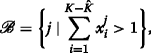(15)

where $$x_{i}^{j} \in \{ 0,1 \}$$, $$\forall i \in \{ 1,2, \cdots, K-\hat {K} \}$$, denotes the link status at node i. $$x_{i}^{j} = 1$$ represents an active transmission at node i and 0 represents waiting or performing energy harvesting by the sub-WET at node i. The link status is expressed as

$$x_{i}^{j}= {\begin{cases} 1 ~& if~\text{node~\textit{i}~in~state~\textit{j}~transmits~data},\\ 0 ~& otherwise.\\ \end{cases}}$$
(16)

In addition, we assume that the waiting time of node i, $$\forall i \in \{ 1,2, \cdots, K-\hat {K} \}$$, is exponentially distributed and λ−1 denotes the mean of the exponential distribution. The probability density function of the waiting time t i is given by

$$f(t_{i};\lambda_{i})= {\begin{cases} \lambda_{i} exp(-\lambda_{i} t_{i}) & if~t_{i} \geq 0,\\ 0 & if~t_{i} < 0,\\ \end{cases}}$$
(17)

where $$\lambda _{i} \in \{ 1,2, \cdots, K-\hat {K} \}$$ denotes the transmitted frames per unit time .

Due to the sensing delay, δT s , experienced by the node i, $$\forall i \in \{ 1,2, \cdots, K-\hat {K} \}$$, the probability, that node i performs the UL WIT within δT s while starting the UL WIT from another node, is expressed as

$$p_{i} \triangleq 1 - exp(-\lambda_{i} \delta T_{s}),$$
(18)

by the memoryless property of the exponential random variable, i.e., CDF . Thus, the rate of transition,, to one of the non-collision states in the Markov chain is defined as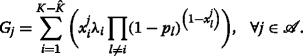(19)

The rate of transition,, to one of the collision states is given by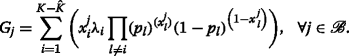(20)

## 4 Problem formulation for the H-DHT protocol

In this section, we study the sum-throughput maximization in the large-scale WPCN based on the H-DHT. Specifically, we aim to maximize the sum-throughput of the DL and UL WIT in the TP, and the UL WIT in the CP, respectively. In the following, we formulate the optimization problem for the TP and the CP.

### 4.1 TDMA period in the H-DHT protocol

In the TP, the H-AP and node i, $$\forall i \in \{ 1,2, \cdots, \hat {K} \}$$, can perform the DL and UL WIT, respectively. $$R_{sum}^{TDMA}$$ denotes the sum-throughput of the H-AP and nodes allocated in the TP and can be expressed as

$$R_{sum}^{TDMA}= \rho_{0}R_{0}+\sum_{i=1}^{\hat{K}} R_{i},$$
(21)

where ρ0 denotes the presence of the DL WIT at the H-AP. If there is information transmission at the H-AP, ρ0 is 1, or if not ρ0 is 0. R0 and R i denote the throughput of the DL WIT and UL WIT, respectively. R0 is obtained by

$$\begin{array}{*{20}l} R_{0}=&\tau_{0_{WIT}}\ln\left({1+\frac{h_{i}\overline{P_{A}}}{\Gamma_{A}\sigma_{A}^{2}}}\right) \\ =&\tau_{0_{WIT}}\ln\left(1+\gamma_{0}\frac{\eta_{A}P_{max}}{\tau_{0_{WIT}}}\right), \end{array}$$
(22)

where $$\gamma _{0} = \frac {h_{i}}{\Gamma _{A}\sigma _{A}^{2}}$$. In addition, R i is obtained by

$$\begin{array}{*{20}l} R_{i}=&\tau_{i}\ln\left({1+\frac{g_{i}P_{i}}{\Gamma\sigma^{2}}}\right) \\ =&\tau_{i}\ln\left(1+\gamma_{i}\frac{\tau_{0_{WET}}+\tau_{0_{CSMA}}}{\tau_{i}}\right),~\forall i \in \{ 1,2, \cdots, K\}, \end{array}$$
(23)

where $$\gamma _{i} = \frac {g_{i}h_{i}\eta _{i}\xi _{i}P_{A}}{\Gamma \sigma ^{2}}$$ and P i from Eq. (10). Γ denotes the signal-to-noise ratio gap from the additive white Gaussian noise channel capacity as a modulation and coding scheme (MCS) use. σ2 represents the noise power at the H-AP. For convenience, we assume ξ i h i P A =1 from Eqs. (8) and (13) in the sequel of this paper without loss of generality.

Consequently, from Eqs. (22) and (23), $$R_{sum}^{TDMA}$$ can be expressed as

$$\begin{array}{*{20}l} R_{sum}^{TDMA}&=\rho_{0}\tau_{0_{WIT}}\ln\left(1+\gamma_{0}^{\prime}\frac{\tau_{0_{WET}}}{\tau_{0_{WIT}}}\right) \\ &+\sum_{i=1}^{\hat{K}}{\tau_{i}\ln\left(1+\gamma_{i}\frac{\tau_{0_{WET}}+\tau_{0_{CSMA}}}{\tau_{i}}\right)} \end{array}$$
(24)

where $$\gamma _{0}^{\prime } = \frac {h_{i}P_{A}}{\Gamma _{A}\sigma _{A}^{2}}$$.

To maximize the sum-throughput in the TP, $$R_{sum}^{TDMA}$$, the optimal time allocation is investigated in the large-scale WPCN. The throughput maximization is then expressed as the following problem:

$$\begin{array}{*{20}l} \text{(P1)}: \indent \max_{\boldsymbol{\tau}}~&R_{sum}^{TDMA}(\tau_{i}) \end{array}$$
(25)
$$\begin{array}{*{20}l} \text{s.t.}~&\tau_{mst} \leq \tau_{i} \end{array}$$
(26)
$$\begin{array}{*{20}l} ~&\sum_{i=0}^{\hat{K}}\tau_{i} \leq T_{t} \end{array}$$
(27)

where Eqs. (26) and (27) correspond to the original constraints from Eqs. (7) and (6), respectively. To solve (P1), we first present the following two lemmas.

### Lemma 3.1

The optimal time allocation of (P1) must satisfy the constraint, Eq. (27), with equality, i.e., $$\sum _{i=0}^{\hat {K}}\tau _{i}^{*}=T_{t}$$.

### Proof

Please refer to Appendix A. □

### Lemma 3.2

The objective function of (P1), Eq. (25), is a concave function of the allocated time for the H-AP and node i, $$\forall i \in \{ 1, 2, \cdots, \hat {K} \}$$, i.e., $$\boldsymbol {\tau }=\left [\tau _{0}, \cdots,\tau _{\hat {K}}\right ]^{T}$$.

### Proof

Please refer to Appendix B. □

### Proposition 3.1

The optimal time allocation of (P1) is

$$\begin{array}{*{20}l} &\tau_{0}^{*} = \frac{1-z^{*}+\mathcal{F} \cdot \mathcal{C}}{1-z^{*}}, \end{array}$$
(28)
$$\begin{array}{*{20}l} &\tau_{i}^{*} =\frac{\gamma_{i} \cdot \mathcal{C}}{1-z^{*}}, \end{array}$$
(29)
$$\begin{array}{*{20}l} &\tau_{0_{WIT}}^{*} = \max_{\boldsymbol{\tau}} \tau_{i}^{*}, \end{array}$$
(30)
$$\begin{array}{*{20}l} &\tau_{0_{WET}}^{*} = \tau_{0}^{*} - \tau_{0_{WIT}}^{*}, \end{array}$$
(31)

where $$z^{*} = (\mathcal {F} -1)/\mathcal {W} \{ (\mathcal {F}-1)/(\exp (1+\rho _{0} \cdot \mathcal {H}) \}$$, $$\mathcal {F} \triangleq \sum _{j=1}^{K} \gamma _{j}$$, and $$\mathcal {W}(\cdot)$$ is the well-known Lambert W-function .

### Proof

Please refer to Appendix C. □

### 4.2 CSMA period in the H-DHT protocol

In the CP, the H-AP performs the sub-WET and node i, $$\forall i \in \{ 1, 2, \cdots, K-\hat {K} \}$$, performs the UL WIT. In Section 2, we describe the system model of the CP as continuous Markov chain model . Now, we optimize the sum-throughput of nodes in the CP by maximizing the probability of being in the non-collision transmission states. For maximizing the probability, the stationary distribution of the continuous Markov chain, denoted by p(xi), is defined as

$$p\left(x^{i}\right) \triangleq \frac{exp(r_{i})}{\sum_{j} exp(r_{j})},$$
(32)

where r i satisfies the detailed balance equation . Eqs. 19 and (20) express r i , which is obtained as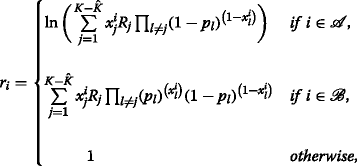(33)

where $$R_{i} \triangleq \frac {\lambda _{i}}{\mu _{i}}$$ denotes the throughput of node i, $$\forall i \in \{ 1, 2, \cdots, K-\hat {K} \}$$. $$\frac {1}{\mu _{i}}$$ denotes the mean transmission length of the information. It is worth noting that for the purpose of exposition, we assume λ=1. Hence, we can interchange the throughput of nodes in the CP and R i from Eq. (23), $$\forall i \in \{ 1, 2, \cdots, K-\hat {K} \}$$.

To quantify the sum-throughput of nodes, a log-likelihood function  is defined as the summation over all the non-collision transmission states, which can be expressed by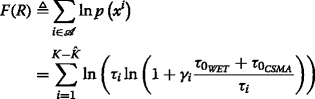(34)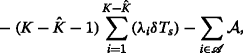(35)

where $$\mathcal {A} \triangleq \ln \sum _{j} exp(r_{j})$$. Then,is defined as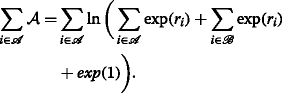(36)

Then, the throughput maximization is then obtained by the following problem:

$$\begin{array}{*{20}l} \text{(P2)}: \indent \max_{\boldsymbol{\tau}}~&F(\tau_{i}) \end{array}$$
(37)
$$\begin{array}{*{20}l} \text{s.t.}~&\tau_{mst} \leq \tau_{i} \end{array}$$
(38)
$$\begin{array}{*{20}l} ~&\sum_{i=1}^{K-\hat{K}} \tau_{i} \leq T_{c} \end{array}$$
(39)

where Eqs. (38) and (39) correspond to the original constraints from Eqs. (7) and (12), respectively. To solve (P2), we first present the following lemma.

### Lemma 3.3

The objective function of (P2) is a concave function of the allocated time for the H-AP and node i, $$\forall i \in \{ 1, 2, \cdots, K-\hat {K} \}$$.

### Proof

Please refer to Appendix D. □

Figure 4 further shows the objective function of (P2) by increasing nodes in the CP, i.e., Eq. (37) is a concave function over the number of nodes in the CP.

### Proposition 3.1

The optimal time allocation of (P2) is

$$\begin{array}{*{20}l} &\tau_{i}^{*} = \frac{\gamma_{i} \mathcal{C}}{q^{*}-1}, \end{array}$$
(40)
$$\begin{array}{*{20}l} &\tau_{0_{CSMA}}^{*} = T_{c} - \sum_{i=1}^{K-\hat{K}} \tau_{i}^{*} \end{array}$$
(41)

where $$q^{*} \triangleq 1+\frac {\gamma _{i} \mathcal {C}}{\tau _{i}^{*}}$$, and q is calculated by the well-known Lambert W-function  as Proposition 3.1.

### Proof

Please refer to Appendix F. □

## 5 Simulation results and discussion

In this section, we present some simulation results to evaluate the performance of the proposed H-DHT protocols in the large-scale WPCN. The harvest-then-transmit (HTT) protocol in  and the adaptive harvest-then-cooperate protocol (AHC) in  are used for the comparison. In the evaluation, the energy harvesting efficiency is assumed to be equal to one for all nodes, i.e., ξ i =1, i{1,2,,N}. The noise power at the receiver, σ2, is assumed to be one, and the energy transmit power of the H-AP for the DL WET, P A , is assumed to be 10 dB. We also assume i.i.d. Rayleigh fading for all channels in the network and the channel power gains of these channels are exponentially distributed, where the mean of the channel power gains is one, i.e., g i =1 and h i =1, i{1,2,,N}, respectively. Although we propose the H-DHT protocol for large-scale WPCN, in which there can be thousands of nodes like IoT or LPWAN networks, we assume that there are a hundred nodes for convenience. Also, we assume sensing delay to check channel state is 0.001 ms, and the network overhead caused by a single SI message is 0.5% in a block time.

Figure 5 shows the effect of the number of nodes to the ratio of the SI transmission period to a block time in the HTT, the AHC, and the proposed H-DHT according to the average probability of generating SI message, P s , respectively. In the proposed H-DHT protocol, the ratio of SI period to block time increases slightly as the number of nodes increases. We can see that the maximum ratio of the SI message transmission period to the block time is different according to the P s , since the P s affects the ratio of the TDMA period to the block time, which decides the maximum receptible number of SI messages. Due to the limitation of the maximum receptible number of SI messages, the increase of the ratio of the SI transmission period to the block time stops when the ratio reaches the maximum value. On the other hand, both the HTT and AHC protocols assume that all nodes in the WPCN transmit SI messages to the H-AP, since we can see that the ratio of the SI message transmission period to the block time increases continuously according to the increase of the number of nodes.

Figure 6a shows the total harvested energy for UL WIT according to the ratio of TDMA period to block time. In the HTT and AHC protocols, a block time is entirely used for TDMA-based WET and WIT. Therefore, the total harvested energy does not change. However, in the proposed H-DHT protocol, nodes harvest energy twice when the H-AP performs a main-WET in the TP and a sub-WET in the CP within a block time. Especially, we assume that the H-AP performs sub-WET when the wireless channel is in idle state in the CP. In general, the channel utilization rate of CSMA protocol for the wireless ad hoc network is very low compared to TDMA protocol . Therefore, in Fig. 6a, we can see that the total harvested energy decreases according to the increase of the ratio of TDMA period to a block time. In addition, Fig. 6b shows that the harvested energy during the CP decreases according to the increase of the ratio of TDMA period to a block time because the sub-WET period decreases.

Figure 7 shows the sum-throughput according to the number of nodes in the HTT, AHC, and the proposed H-DHT. As we can see in the Fig. 7, the throughput of both the HTT and AHC protocols decreases when the number of nodes increases, because of the network overhead caused by SI transmission. However, the sum-throughput of the H-DHT protocol increases according to the number of nodes, because the H-DHT protocol permit only the nodes, which require reliable data transmission, to send SI message to the H-AP. Therefore, the network overhead caused by SI message transmission is relatively low compared to the HTT and AHC protocols. Also, the result shows that the sum-throughput increases when the P s increases, because high P s indicates that channel utilization rate increases.

In addition, the evaluation results show that the proposed H-DHT protocol outperforms the conventional protocol, HTT and AHC, in terms of channel allocation. In the H-DHT protocol, only the nodes which want to obtain a time slot of TP generate SI message. Therefore, in Fig. 5, we can see that the network overhead caused by SI is decreased in the H-DHT compared to the conventional protocols, HTT and AHC. As a result, channel resource for WIT/WET is increased. Figure 6, also, shows that the proposed H-DHT protocol outperforms the conventional protocols, HTT and AHC in terms of channel allocation. The H-DHT protocol performs sub-WET at space holes in the CT, so there is no wasted time. As a result, in Fig. 6a, we can see that the nodes in the proposed H-DHT protocol harvest more energy compared to nodes in the HTT and AHC, in most cases according to ratio of TDMA period to block time.

## 6 Conclusions

This paper proposes a novel protocol for a large-scale WPCN, namely hybrid multiple access-based dual harvest-then-transmit (H-DHT). The proposed H-DHT protocol has a hybrid multiple access structure which employs both TDMA and CSMA, in order to manage different traffic patterns and requirements depending on applications. Thus, the H-DHT protocol provides transmission reliability for transmitting information of nodes by TDMA. Furthermore, we propose a novel wireless energy transfer method, named dual-WET, which improves the channel utilization and increases the amount of harvested energy at nodes. Specifically, from dual-WET, the nodes can perform energy harvesting at space holes in CSMA. In addition, we describe the novel procedure of SI message transmission between the H-AP and nodes to reduce network overhead caused by numerous SI messages. For the consideration, simulation results reveal that the H-DHT outperforms the existing WPCN protocols in a large-scale network, in terms of sum-throughput, SI transmission overhead, and an amount of harvested energy at nodes.

There are some research issues remained to improve the proposed system model for future work. Full-duplex WPCN models can be consider to the TP in order to improve harvested energy from main-WET, where the self-interference is fully prevented. Additionally, we will expend the QoS in terms of receiving information as considering the energy efficiency.

## 7 \thelikesection Appendix

### 7.1 \thelikesubsection Appendix A: Proof of Lemma 3.1

This can be proved by contradiction. Suppose $$\boldsymbol {\tau }^{\prime }=\left [\tau _{0}^{\prime }, \cdots,\tau _{\hat {K}}^{\prime }\right ]^{T}$$ is an optimal solution of (P1), and it satisfies that $$\sum _{i=0}^{\hat {K}}\tau _{i}^{\prime }<T_{t}$$. It follows that $$\tau _{0}^{\prime }<T_{t}-\sum _{i=1}^{\hat {K}}\tau _{i}^{\prime }$$. The objective function given in Eq. (25) is a monotonic increasing function with respect to τ0. Thus, the value of Eq. (25) under the vector $$\left [\tau _{0}^{\prime }, \cdots,\tau _{\hat {K}}^{\prime }\right ]^{T}$$ is larger than that under τ. This contradicts with our presumption. Thus, the optimal τ must satisfy $$\sum _{i=0}^{\hat {K}}\tau _{i}^{*}=T_{t}$$.

This completes the proof of Lemma 3.1.

### 7.2 \thelikesubsection Appendix B: Proof of Lemma 3.2

According to , a function is concave if its Hessian is negative semidefinite. Thus, to show $$R_{sum}^{TMDA}(\boldsymbol {\tau })$$ is a concave function of τ, we denote the Hessian of $$R_{sum}^{TDMA}(\boldsymbol {\tau })$$ by H i and demonstrate that H i is a negative semidefinite. For any given real vector ν=[ν0,,ν K ]T, it follows that

$$\boldsymbol{\nu}^{T}\mathit{\boldsymbol{H}_{i}}\boldsymbol{\nu} \leq 0,$$
(42)

where the inequality follows from the fact that τ mst τ i . Thus, H i is negative semidefinite. Therefore, $$R_{sum}^{TDMA}(\boldsymbol {\tau })$$ is a concave function of $$\boldsymbol {\tau }=\left [\tau _{0}, \cdots,\tau _{\hat {K}}\right ]^{T}$$.

This completes the proof of Lemma 3.2.

### 7.3 \thelikesubsection Appendix C: Proof of Proposition 3.1

$$\begin{array}{*{20}l} \mathcal{L}_{sum}(\boldsymbol{\tau}, \lambda)=&R_{sum}^{TDMA}(\boldsymbol{\tau}) -\lambda\left(\sum_{i=0}^{\hat{K}}\tau_{i}-T_{t}\right), \end{array}$$
(43)

where λ≥0 denotes the Lagrange multiplier with the constraint in Eq. (27). The dual function of (P1) is thus given by

$$\mathcal{G}(\lambda) = \min_{\boldsymbol{\tau} \in \mathcal{D}} \mathcal{L}_{sum}(\boldsymbol{\tau}, \lambda),$$
(44)

where $$\mathcal {D}$$ is the feasible set of τ specified by Eqs. (26) and (27). Thus, strong duality holds for this problem thanks to the Slater’s condition.

Since (P1) is a convex optimization problem for which the strong duality holds, the Karush-Kuhn-Tucker (KKT) conditions are both necessary and sufficient for the global optimality of (P1), which are given by

$$\sum_{i=0}^{\hat{K}}\tau_{i}^{*} \leq T_{t},$$
(45)
$$\lambda^{*} \left(\sum_{i=0}^{\hat{K}}\tau_{i}^{*} - T_{t} \right) = 0,$$
(46)
$$\frac{\partial}{\partial\tau_{i}}R_{sum}^{TDMA}(\boldsymbol{\tau}^{*})-\lambda^{*}=0,~~~\forall i \in \{ 0,1, \cdots, \hat{K} \},$$
(47)

where $$\tau _{i}^{*}$$ and λ denote the optimal primal and dual solutions of (P1), respectively. It can be easily be verified that $$\sum _{i=0}^{\hat {K}}\tau _{i}^{*} = T_{t}$$ must hold for (P1) and thus from Eq. (27) without loss of generality, we assume λ>0. It is worth noting that for convenience, we normalize T t as 1 in the sequel.

$$\begin{array}{*{20}l} \rho_{0}\Bigg{\{} \ln\left(1+\gamma_{0}^{\prime} \mathcal{B}\right) &+ (1-\mathcal{B}) \Bigg{(} \frac{\gamma_{0}^{\prime}}{1+ \gamma_{0}^{\prime} \mathcal{B}} \Bigg{)} \Bigg{\}} \\ &+ \sum_{i=1}^{\hat{K}} \frac{\gamma_{i}}{1+ \gamma_{i} \frac{\mathcal{C}}{\tau_{i}}} =\lambda^{*}, \end{array}$$
(48)

where $$\mathcal {B} \triangleq \frac {\tau _{0} - \tau _{0_{WIT}}}{\tau _{0} - \tau _{0_{WET}}}$$, $$\mathcal {C} \triangleq \tau _{0} - \tau _{0_{WIT}} + \tau _{0_{CSMA}}$$, and $$\mathcal {H} \triangleq \ln (1+\gamma _{0}^{\prime } \mathcal {B}) + (1-\mathcal {B}) \Big {(} \frac {\gamma _{0}^{\prime }}{1+ \gamma _{0}^{\prime } \mathcal {B}} \Big {)}$$.

$$\mathcal{X}\left(\gamma_{i} \frac{\mathcal{C}}{\tau_{i}}\right) = \lambda^{*},~~~1\leq i \leq \hat{K},$$
(49)

where $$\mathcal {X}(\alpha)\triangleq \ln (1+\alpha) - \frac {\alpha }{1+\alpha },~\alpha \geq 0$$.

Given 1≤i, $$j \leq \hat {K}$$, from Eq. (48) we have

$$\mathcal{X}\left(\gamma_{i} \frac{\mathcal{C}}{\tau_{i}}\right) = \mathcal{X}\left(\gamma_{j} \frac{\mathcal{C}}{\tau_{j}}\right),~~~i \neq j.$$
(50)

It can be easily shown that $$\mathcal {X}(\alpha)$$ is a monotonically increasing function of α≥0 since $$\frac {d \mathcal {X}(\alpha)}{d\alpha } \geq 0$$ for α≥0. Therefore, equality in Eq. (50) holds if and only if $$\gamma _{i} \frac {\mathcal {C}}{\tau _{i}} = \gamma _{j} \frac {\mathcal {C}}{\tau _{j}}$$, 1≤i, $$j \leq \hat {K}$$, i.e.,

$$\frac{\gamma_{1}}{\tau_{1}} = \frac{\gamma_{2}}{\tau_{2}} = \cdots=\frac{\gamma_{\hat{K}}}{\tau_{\hat{K}}}=D.$$
(51)

From Lemma 3.1 and $$\tau _{j}^{*} = \frac {\gamma _{j}}{\gamma _{i}}\tau _{i}^{*}$$, $$\tau _{i}^{*}$$ can be expressed as

$$\begin{array}{*{20}l} \tau_{i}^{*} = \left(1-\tau_{0}^{*}\right) \frac{\gamma_{i}}{\sum_{j=1}^{\hat{K}} \gamma_{j}}, \end{array}$$
(52)

where $$\mathcal {F}=\sum _{j=1}^{\hat {K}} \gamma _{j}$$. In addition, it follows from Eqs. (47), (51), and (52) that

$$\begin{array}{*{20}l} \ln \left(1+\frac{\mathcal{F} \cdot \mathcal{C}}{1-\tau_{0}^{*}}\right) - \frac{\frac{\mathcal{F} \cdot \mathcal{C}}{1-\tau_{0}^{*}}}{1+ \frac{\mathcal{F} \cdot \mathcal{C}}{1-\tau_{0}^{*}}} = \rho_{0} \cdot \mathcal{H} + \frac{\mathcal{F}}{1+ \frac{\mathcal{F} \cdot \mathcal{C}}{1-\tau_{0}^{*}} }. \end{array}$$
(53)

We can modify Eq. (53) as

$$\begin{array}{*{20}l} z \ln z - z(1 + \rho_{0} \cdot \mathcal{H}) - \mathcal{F} + 1 = 0, \end{array}$$
(54)

where $$z=1+ \frac {F \cdot C}{1-\tau _{0}}$$. Therefore, the optimal time allocation is given by

$$\begin{array}{*{20}l} \tau_{0}^{*} = \frac{1-z^{*}+\mathcal{F} \cdot \mathcal{C}}{1-z^{*}}. \end{array}$$
(55)

From Lemma 3.1, τ i is obtained as

$$\begin{array}{*{20}l} \tau_{i}^{*} =\frac{\gamma_{i} \cdot \mathcal{C}}{1-z^{*}}, \end{array}$$
(56)

where z is calculated by the well-known Lambert W-function  as

$$\begin{array}{*{20}l} z^{*} = \frac{\mathcal{F} -1}{\mathcal{W}\bigg{(}\frac{\mathcal{F}-1}{ \exp(1+\rho_{0} \cdot \mathcal{H})} \bigg{)}}. \end{array}$$
(57)

$$\tau _{0_{WIT}}^{*}$$ is obtained as

$$\begin{array}{*{20}l} \tau_{0_{WIT}}^{*} = \max_{\boldsymbol{\tau}} \tau_{i}^{*}, \end{array}$$
(58)

since we assume that γ i =1, and

$$\tau_{0_{WET}}^{*} = \tau_{0} - \tau_{0_{WIT}}.$$
(59)

This thus proves Proposition 3.1.

### 7.4 \thelikesubsection Appendix D: Proof of Lemma 3.3

We may note that the proof of Lemma 3.3 is essentially equal to Lemma 3.2.

### 7.5 \thelikesubsection Appendix F: Proof of Proposition 3.2

$$\begin{array}{*{20}l} \mathcal{L}_{sum}(\boldsymbol{\tau}, \lambda)=&F(\boldsymbol{\tau}) -\lambda\left(\sum_{i=1}^{K-\hat{K}}\tau_{i}-1\right) \end{array}$$
(60)

where λ≥0 denotes the Lagrange multiplier with the constraint in Eq. (39). The dual function of (P2) is thus given by

$$\mathcal{G}(\lambda) = \min_{\boldsymbol{\tau} \in \mathcal{D}} \mathcal{L}_{sum}(\boldsymbol{\tau}, \lambda)$$
(61)

where $$\mathcal {D}$$ is the feasible set of τ specified by Eqs. (38) and (39). Thus, strong duality holds for this problem thanks to the Slater’s condition.

Since (P2) is a convex optimization problem for which the strong duality holds, the Karush-Kuhn-Tucker (KKT) conditions are both necessary and sufficient for the global optimality of (P2), which are given by

$$\sum_{i=1}^{K-\hat{K}}\tau_{i}^{*} \leq T_{c}$$
(62)
$$\lambda^{*} \Bigg{(} \sum_{i=1}^{K-\hat{K}}\tau_{i}^{*} - T_{c} \Bigg{)} = 0$$
(63)
$$\frac{\partial}{\partial\tau_{i}}F(\boldsymbol{\tau}^{*})-\lambda^{*}=0,~~~\forall i \in \{ 1,2, \cdots, K-\hat{K}\}$$
(64)

where $$\tau _{i}^{*}$$ and λ denote the optimal primal and dual solutions of (P2), respectively. It is worth noting that for convenience, we normalize T c as 1 in the sequel. Then, from Eq. (64), it follows that

$$\begin{array}{*{20}l} \frac{1}{\tau_{i}^{*}} - \frac{\gamma_{i} \mathcal{C}}{\left(1+\gamma_{i} \frac{\mathcal{C}}{\tau_{i}^{*}}\right) \ln\left(1+\gamma_{i} \frac{\mathcal{C}}{\tau_{i}^{*}}\right)} - \mathcal{J} = \lambda^{*} \end{array}$$
(65)

where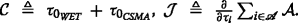. First, consider the case of λ>0, which corresponds to $$\sum _{i=i}^{K-\hat {K}\tau _{i}^{*}} = 1$$ from Eq. (39). Given 1≤i, $$j \geq K-\hat {K}$$ in the (65), we have equal result at i=j, since the (65) is monotonic function, i.e., $$\tau _{j}^{*} = \frac {\gamma _{j}}{\gamma _{i}}\tau _{i}^{*}$$ and $$\tau _{i}^{*} = \frac {\gamma _{i}}{\sum _{j=1}^{K-\hat {K}}}\gamma _{j}$$ same as (51) and (52).

Next, for the case of λ=0, the left side of (65) is zero. We can modify (65) as

$$\begin{array}{*{20}l} q\ln q \left(\frac{q-1}{(q-1)\tau_{i}}-\mathcal{J}\right) = (q-1)\tau_{i} \end{array}$$
(66)

where $$q \triangleq 1+\frac {\gamma _{i} \mathcal {C}}{\tau _{i}}$$. Therefore, optimal time of the node i is obtained as

$$\begin{array}{*{20}l} \tau_{i}^{*} = \frac{\gamma_{i} \mathcal{C}}{q^{*}-1} \end{array}$$
(67)

where q is calculated by the Lambert W-function  as Proposition 3.1.

This completes the proof of Proposition 3.2.

## References

1. K Wang, Y Wang, Y Sun, S Guo, J Wu, Green industrial internet of things architecture: an energy-efficient perspective. IEEE Commun. Mag.54(12), 48–54 (2016).

2. Standardization of NB-IOT Completed. http://www.3gpp.org/news-events/3gpp-news/1785-nb_iot_complete. Accessed 28 December 2017.

3. N Sornin, M Luis, T Eirich, T Kramp, O Hersent, LoRa Alliance. LoRaWAN Specification (LoRa Alliance, San Ramon, 2015). https://www.rs-online.com/designspark/rel-assets/ds-assets/uploads/knowledge-items/application-notes-for-the-internet-of-things/LoRaWAN%2520Specification%25201R0.pdf.

4. O Ozel, K Tutuncuoglu, J Yang, S Ulukus, A Yener, Transmission with energy harvesting nodes in fading wireless channels: optimal policies. IEEE J. Sel. Areas Commun.29:, 1732–1743 (2011).

5. CK Ho, R Zhang, Optimal energy allocation for wireless communications with energy harvesting constraints. IEEE Trans. Sig. Process.60:, 4808–4818 (2012).

6. S Bi, CK Ho, R Z, Wireless powered communication: opportunities and challenges. IEEE Commun. Mag.53: (2015).

7. X Zhou, R Zhang, CK Ho, Wireless information and power transfer: architecture design and rate-energy tradeoff. IEEE Trans. Commun.61:, 4757–4763 (2013).

8. L Liu, R Zhang, KC Chua, Wireless information and power transfer: a dynamic power splitting approach. IEEE Trans. Commun.61:, 3990–4001 (2013).

9. R Zhang, CK Ho, Mimo broadcasting for simultaneous wireless information and power transfer. IEEE Trans. Wirel. Commun.12:, 1989–2001 (2013).

10. K Huang, E Larsson, Simultaneous information and power transfer for broadband wireless systems. IEEE Trans. Signal Process.61:, 5972–5986 (2013).

11. H Ju, R Zhang, Throughput maximization in wireless powered communication networks. IEEE Trans. Wirel. Commun.13:, 418–428 (2014).

12. H Ju, R Zhang, Optimal resource allocation in full-duplex wireless-powered communication network. IEEE Trans. Commun.62:, 3528–3540 (2014).

13. X Kang, CK Ho, S Sun, Full-duplex wireless-powered communication network with energy casuality. IEEE Trans. Wirel. Commun.14:, 5539–5551 (2015).

14. X Zhou, R Zhang, CK Ho, Wireless information and power transfer in multiuser ofdm systems. IEEE Trans. Wirel. Commun.13:, 2282–2294 (2014).

15. Y Cheng, P Fu, Y Chang, B Li, X Yuan, Joint power and time allocation in full-duplex wireless powered communication networks. Mob. Inf. Syst.2016:, 1–15 (2016).

16. SH Lee, R Zhang, KB Huang, Opportunistic wireless energy harvesting in cognitive radio networks. IEEE Trans. Wirel. Commun.12:, 4788–4799 (2013).

17. SH Lee, R Zhang, Cognitive wireless powered network: spectrum sharing models and throughput maximization. IEEE Trans. Cogn. Commun. Netw.1:, 335–346 (2015).

18. Q Yao, A Huang, H Shan, T Quek, W Wang, Delay-aware wireless powered communication networks—energy balancing and optimization. IEEE Trans. Wirel. Commun.15:, 5272–5286 (2016).

19. Q Yao, A Huang, H Shan, T Quek, W Wang, Adaptive harvest-then-cooperate: delay-aware wireless powered communication networks. 2016 IEEE 17th Int. Workshop Sig. Process. Adv. Wirel. Commun (SPAWC). 15:, 1–5 (2016).

20. E Karapistoli, FN Pavlidou, I Gragopoulos, I Tsetsinas, An overview of the IEEE 802.15.4a standard. IEEE Commun. Mag.48:, 47–53 (2010).

21. L Jiang, H Tian, Z Xing, K Wang, K Zhang, S Maharjan, S Gjessing, Y Zhang, Social-aware energy harvesting device-to-device communications in 5g networks. IEEE Wirel. Commun.23(4), 20–27 (2016).

22. D Zeng, J Zhang, S Guo, L Gu, K Wang, Take renewable energy into cran toward green wireless access networks. IEEE Netw.31(4), 62–68 (2017).

23. Y Wu, Y Chen, J Tang, D So, Z Xu, I C, P Ferrand, J Gorce, C Tang, P Li, K Feng, L Wang, K Borner, L Thiele, Green transmission technologies for balancing the energy efficiency and spectrum efficiency trade-off. IEEE Commun. Mag.52(11), 112–120 (2014).

24. H Zhang, H Liu, J Cheng, VCM Leung, Downlink energy efficiency of power allocation and wireless backhaul bandwidth allocation in heterogeneous small cell networks. IEEE Trans. Commun.PP(99), 1–1 (2017). https://doi.org/10.1109/TCOMM.2017.2763623. http://ieeexplore.ieee.org/document/8070320/citations.

25. H Zhang, S Huang, C Jiang, K Long, V Leung, H Poor, Energy efficient user association and power allocation in millimeter-wave-based ultra dense networks with energy harvesting base stations. IEEE J. Sel. Areas Commun.35(9), 1936–1947 (2017).

26. H Zhang, C Jiang, N Beaulieu, X Chu, X Wang, T Quek, Resource allocation for cognitive small cell networks: a cooperative bargaining game theoretic approach. IEEE Trans. Wirel. Commun.14(6), 3481–3493 (2015).

27. H Zhang, Y Nie, J Cheng, V Leung, A Nallanathan, Sensing time optimization and power control for energy efficient cognitive small cell with imperfect hybrid spectrum sensing. IEEE Trans. Wirel. Commun.16(2), 730–743 (2017).

28. H Zhang, C Jiang, X Mao, H Chen, Interference-limited resource optimization in cognitive femtocells with fairness and imperfect spectrum sensing. IEEE Trans. Veh. Technol.65(3), 1761–1771 (2016).

29. J Tang, D So, E Alsusa, K Hamdi, A Shojaeifard, Resource allocation for energy efficiency optimization in heterogeneous networks. IEEE J Sel. Areas Commun.33(10), 2104–2117 (2015).

30. S Subramanian, EL Pasiliao, JM Shea, MM Carvalho, WE Dixon, in IEEE MILITARY COMMUNICATIONS CONFERENCE, 2012. Throughput maximization in csma networks with collisions, (2012), pp. 1–6. https://doi.org/10.1109/MILCOM.2012.6415717. http://ieeexplore.ieee.org/document/6415717/citations.

31. DF Schmidt, E Makalic, Universal models for the exponential distribution. IEEE Trans. Inf. Theory.55:, 3087–3090 (2009).

32. LambertW-Function. http://mathworld.wolfram.com/LambertW-Function.html. Accessed 28 Dec 2017.

33. L Jiang, J Walrand, A distributed CSMA algorithm for throughput and utility maximization in wireless networks. IEEE/ACM Trans. Networking.18:, 960–972 (2010).

34. KP Kelly, Reversibility and Stochastic Networks (Wiley, Chichester, 1979).

35. SM Stigler, Statistics on the Table: The History of Statistical Concepts and Methods (Harvard University Press, Cambridge, 1999).

36. T-J Lee, HR Lee, MY Chung, Mac throughput limit analysis of slotted csma/ca in ieee 802.15.4 wpan. IEEE Commun. Lett.10:, 561–563 (2006).

37. SP Boyd, Convex Optimization (Cambridge University Press, Cambridge, 2004).

## Acknowledgements

The SC, KL, and BK would like to acknowledge the encouragements and suggestions provided by Prof. Inwhee Joe during this research.

Not applicable.

### Availability of data and materials

The datasets of this paper are included within its additional files.

## Author information

Authors

### Contributions

SC and KL propose and analyze the H-DHT protocol based in large-scale WPCN. BK modified the English expressions. IJ organizes the whole paper as well as the proofreading. All authors read and approved the final manuscript.

### Corresponding author

Correspondence to Inwhee Joe.

## Ethics declarations

Not applicable.

Not applicable.

### Competing interests

The authors declare that they have no competing interests.

### Publisher’s Note

Springer Nature remains neutral with regard to jurisdictional claims in published maps and institutional affiliations.

## Rights and permissions